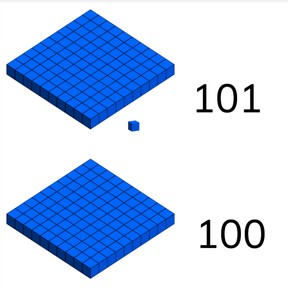Introduction to numbers above 100

# Introduction to numbers above 100

Introduction to numbers above 1008,000 schools use Gynzy92,000 teachers use Gynzy1,600,000 students use Gynzy

## General

Students are introduced to numbers between 100 and 300. They learn how these numbers are built and where they appear on the number line.

2.NBT.A.1

## Relevance

Knowing numbers between 100 and 300 is needed to start doing working with higher numbers.

## Introduction

Start by looking for the biggest number with the students. Have students explain how they decided or figured out that a number is the biggest. First look at the tens and then look at the ones. Repeat this with the different parts of the number 87. Say the word, explain the tens, the ones, and the neighbor numbers. Ask if students know any numbers above 100. Discuss these numbers. Do numbers stop at 100? Who knows how they go on?

## Development

Tell the class that the numbers between 100 and 200 are exactly one hundred. A hundred is a group of 100. On the interactive whiteboard this is visualized by a block of MAB-blocks. You can also show this in the class. If you count on with ones, represented by blocks of 1, you get 101, 102, 103, etc. Ask students to count on. If you count on with blocks of 10, you get 110, 120, 130, etc. Ask students to count on. Show, visually, with MAB blocks what the difference between 24, 124, and 224 are. Demonstrate that they always each have 100 more than then previous number. Practice with creating 213 in MAB blocks. First use the hundreds, then the tens, and then the ones. Students can do this at their desks with real bocks (individually, in pairs, or in groups). Tell that this number has 2 hundred, 1 ten, and 3 ones, using the HTO table. Then you read a news article. Place the number 187 in context. Ask the students: "what else can come in a group of 187?" (number of students at school, books in the school library, colored pencils in class, etc). Then discuss the HTO-table and neighbor numbers of 187. Then set two numbers in the HTO-table. Ask which one is bigger and how you can see this. 153 and 135. In this case, you look at the tens. 153 has more tens. Ask if they can jump between the neighbor hundreds of 153 with a visual number line. Practice skip counting in 10s from 100 to 200. You should as a class hear "one-hundred ten, one-hundred twenty, one-hundred thirty..." starting with that one-hundred every time. Can the students count further? What comes after 200?

Check that students have been introduced to numbers bigger than 100 by asking the following questions:
- Where do you see numbers bigger than 100?
- How do you know which number is bigger? What do you look at first? (first hundreds, then thens, then ones)

## Guided Practice

Students first practice counting backwards in steps of 1. Then they are asked of the value of 1 in a number.

## Closing

Emphasize that it is necessary to know how numbers bigger than 100 are put together, so you can work with numbers later. Check that students can name different aspects of a number like you did in the start of the lesson (naming tens, ones, neighbor numbers). But do it this time with a number above 100.

## Teaching Tip

For students who find this challenging, support their learning by using as much visual support as possible in the form of MAB blocks and other manipulatives. In this way the hundreds, tens, and ones become more visually understandable. Students can also have trouble mixing up the numbers. Repeat often that you first write the hundreds, then the tens, and finally the ones. You can use the HTO-table.

## Instruction materials

MAB blocks (100, 10, 1).

### The online teaching platform for interactive whiteboards and displays in schools

• Save time building lessons

• Manage the classroom more efficiently

• Increase student engagement